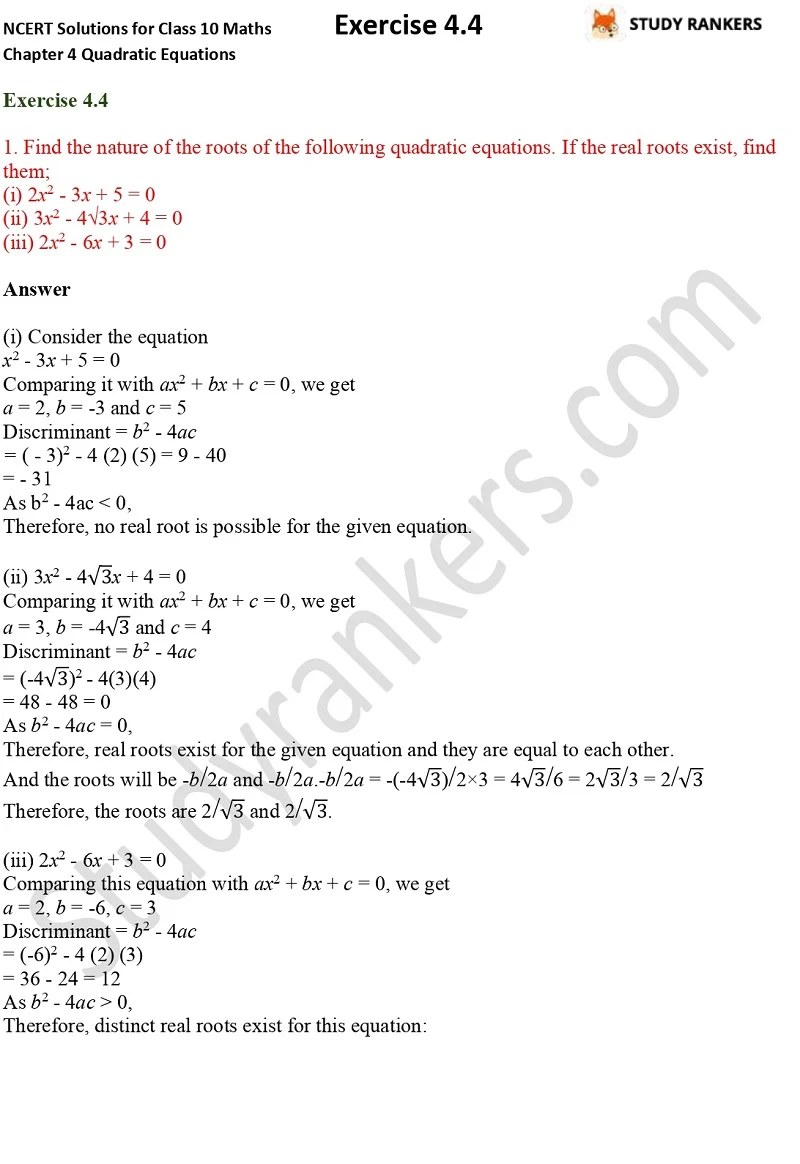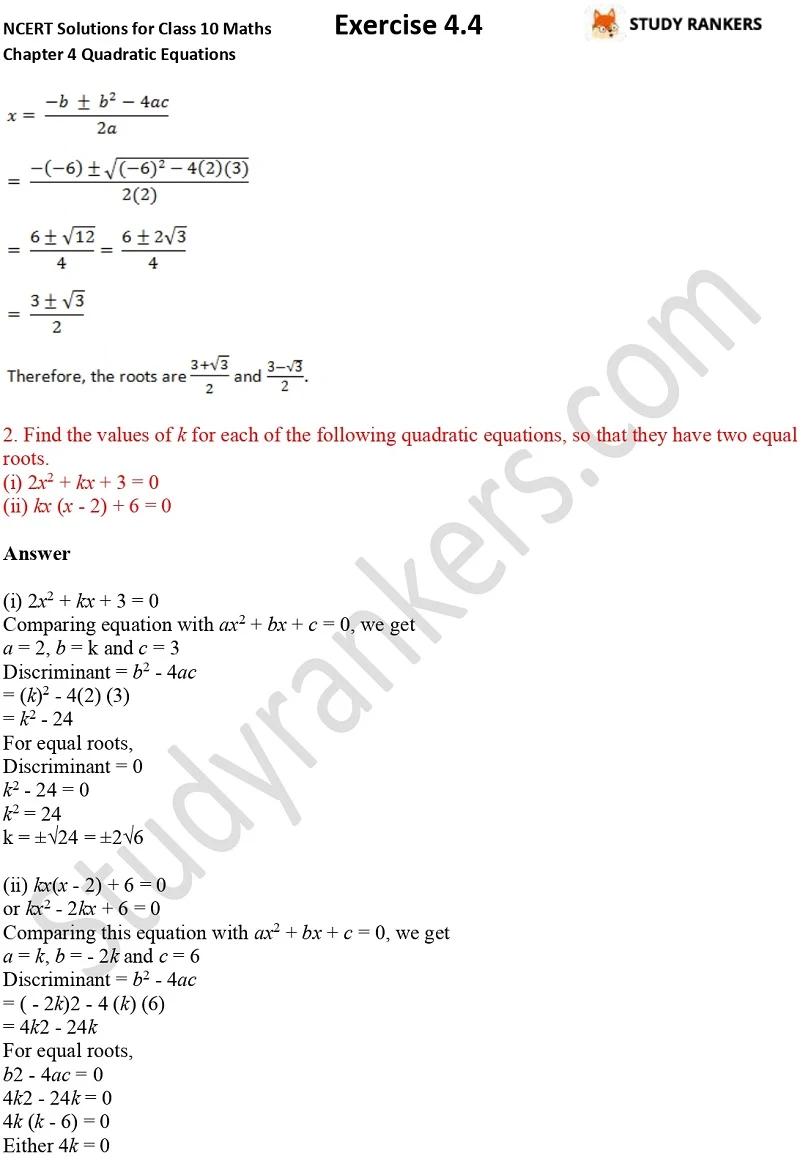## NCERT Solutions for Class 10 Maths Chapter 4 Quadratic Equations Exercise 4.4

If you want NCERT Solutions for Class 10 Maths then you're at right place. In this page, you will find NCERT Solutions for Chapter 4 Quadratic Equations Exercise 4.4. You must understand the basic concepts of solving questions that will boost your morale. These NCERT Solutions are updated according to the latest NCERT textbooks. Whenever you find any problem difficult, you can take help from our study guides.

There are five questions in the exercise 4.4. You have to find the nature of the roots of the given quadratic equations while some questions are word problems which you have to solve by applying formula of quadratic equations.